﻿ 分支理论在肿瘤增长模型中的应用

# 分支理论在肿瘤增长模型中的应用Application of Bifurcation Theory in Tumor Growth Model

Abstract: The paper does qualitative research on a four-dimensional tumor growth model by using center manifolds theory and local bifurcation theory and proves the existence of Transcritical bifurcation and Hopf bifurcation theoretically. It turns out that the patient will recover from the tumor within some time by self-immune system if the tumor growth rate could drop below the threshold with the treatment.

1. 引言

2. 理论分析

2.1. 模型

$\left\{\begin{array}{l}\stackrel{˙}{x}={\rho }_{1}x\left(1-x\right)-{\alpha }_{13}xz\\ \stackrel{˙}{y}=\frac{{\rho }_{2}yz}{1+z}-{\alpha }_{23}yz-{\delta }_{2}y+{\alpha }_{24}yw\\ \stackrel{˙}{z}={\rho }_{3}z\left(1-z\right)-{\alpha }_{31}zx-{\alpha }_{32}zy+\frac{{\alpha }_{34}zw}{1+w}\\ \stackrel{˙}{w}=\frac{{\rho }_{4}wz}{1+z}-{\delta }_{4}w\end{array}\text{}$ (1)

2.2. 平衡点的存在性

$\left\{\begin{array}{l}{\rho }_{1}{x}_{1}\left(1-{x}_{1}\right)-{\alpha }_{13}{x}_{1}{x}_{3}=0\\ \frac{{\rho }_{2}{x}_{2}{x}_{3}}{1+{x}_{3}}-{\alpha }_{23}{x}_{2}{x}_{3}-{\delta }_{2}{x}_{2}+{\alpha }_{24}{x}_{2}{x}_{4}=0\\ {\rho }_{3}{x}_{3}\left(1-{x}_{3}\right)-{\alpha }_{31}{x}_{3}{x}_{1}-{\alpha }_{32}{x}_{3}{x}_{2}+\frac{{\alpha }_{34}{x}_{3}{x}_{4}}{1+{x}_{4}}=0\text{}\\ \frac{{\rho }_{4}{x}_{4}{x}_{3}}{1+{x}_{3}}-{\delta }_{4}{x}_{4}=0\text{}\end{array}$ (2)

${P}_{0}\left(0,0,0,0\right)$, ${P}_{1}\left(0,0,1,0\right)$, ${P}_{2}\left(1,0,0,0\right)$, ${P}_{3}\left(0,0,b,\frac{{\rho }_{3}\left(b-1\right)}{{\alpha }_{34}-{\rho }_{3}\left(b-1\right)}\right)$,

${P}_{4}\left(0,\frac{{\rho }_{3}}{{\alpha }_{32}}\left(1-{c}_{1}\right),{c}_{1},0\right)$, ${P}_{5}\left(0,\frac{{\rho }_{3}}{{\alpha }_{32}}\left(1-{c}_{2}\right),{c}_{2},0\right)$, ${P}_{6}\left(\frac{{\rho }_{3}\left({\alpha }_{13}-{\rho }_{1}\right)}{{\alpha }_{31}{\alpha }_{13}-{\rho }_{1}{\rho }_{3}},0,\frac{{\rho }_{1}\left({\alpha }_{31}-{\rho }_{3}\right)}{{\alpha }_{31}{\alpha }_{13}-{\rho }_{1}{\rho }_{3}},0\right)$,

${P}_{7}\left(0,\frac{1}{{\alpha }_{32}}\left({\rho }_{3}\left(1-b\right)+\frac{{\alpha }_{34}d}{1+d}\right),b,d\right)$, ${P}_{8}\left(1-\frac{{\alpha }_{13}}{{\rho }_{1}}b,0,b,\frac{{\alpha }_{31}\left(1-\frac{{\alpha }_{13}}{{\rho }_{1}}b\right)-{\rho }_{3}\left(1-b\right)}{{\rho }_{3}\left(1-b\right)-{\alpha }_{31}\left(1-\frac{{\alpha }_{13}}{{\rho }_{1}}b\right)}\right)$,

${P}_{9}\left(1-\frac{{\alpha }_{13}}{{\rho }_{1}}{c}_{1},\frac{1}{{\alpha }_{32}}\left[{\rho }_{3}\left(1-{c}_{1}\right)-{\alpha }_{31}\left(1-\frac{{\alpha }_{13}}{{\rho }_{1}}{c}_{1}\right)\right],{c}_{1},0\right)$,

${P}_{10}\left(1-\frac{{\alpha }_{13}}{{\rho }_{1}}{c}_{2},\frac{1}{{\alpha }_{32}}\left[{\rho }_{3}\left(1-{c}_{2}\right)-{\alpha }_{31}\left(1-\frac{{\alpha }_{13}}{{\rho }_{1}}{c}_{2}\right)\right],{c}_{2},0\right)$,

${P}_{11}\left(1-\frac{{\alpha }_{13}}{{\rho }_{1}}b,\frac{{\rho }_{3}}{{\alpha }_{32}}\left(1-b\right)-{\alpha }_{31}\left(1-\frac{{\alpha }_{13}}{{\rho }_{1}}b\right)+\frac{{\alpha }_{34}d}{1+d},b,d\right)$.

${c}_{1}=\frac{{\rho }_{2}-{\alpha }_{23}-{\delta }_{2}-\sqrt{{\left({\rho }_{2}-{\alpha }_{23}-{\delta }_{2}\right)}^{2}-4{\alpha }_{23}{\delta }_{2}}}{2{\alpha }_{23}}$ ;

${c}_{2}=\frac{{\rho }_{2}-{\alpha }_{23}-{\delta }_{2}+\sqrt{{\left({\rho }_{2}-{\alpha }_{23}-{\delta }_{2}\right)}^{2}-4{\alpha }_{23}{\delta }_{2}}}{2{\alpha }_{23}}$.

2.3. 平衡点的个数，类型和稳定性

$\begin{array}{l}{\rho }_{1}=0.518,\text{\hspace{0.17em}}\text{\hspace{0.17em}}{\rho }_{2}=4.5,\text{\hspace{0.17em}}\text{\hspace{0.17em}}{\rho }_{4}=0.86,\text{\hspace{0.17em}}\text{\hspace{0.17em}}{\delta }_{2}=0.5,\text{\hspace{0.17em}}\text{\hspace{0.17em}}{\delta }_{4}=1/11,\text{\hspace{0.17em}}\text{\hspace{0.17em}}{\alpha }_{13}=1.5,\\ {\alpha }_{23}=0.2,\text{\hspace{0.17em}}\text{\hspace{0.17em}}{\alpha }_{24}=0.3,\text{\hspace{0.17em}}\text{\hspace{0.17em}}{\alpha }_{31}=1,\text{\hspace{0.17em}}\text{\hspace{0.17em}}{\alpha }_{32}=2.5,\text{\hspace{0.17em}}\text{\hspace{0.17em}}{\alpha }_{34}=0.75\end{array}$

$\left\{\begin{array}{l}{\stackrel{˙}{x}}_{1}=0.518{x}_{1}\left(1-{x}_{1}\right)-1.5{x}_{1}{x}_{3}\\ {\stackrel{˙}{x}}_{2}=\frac{4.5{x}_{2}{x}_{3}}{1+{x}_{3}}-0.2{x}_{2}{x}_{3}-0.5{x}_{2}+0.3{x}_{2}{x}_{4}\\ {\stackrel{˙}{x}}_{3}={\rho }_{3}{x}_{3}\left(1-{x}_{3}\right)-{x}_{3}{x}_{1}-2.5{x}_{3}{x}_{2}+\frac{0.75{x}_{3}{x}_{4}}{1+{x}_{4}}\\ {\stackrel{˙}{x}}_{4}=\frac{0.86{x}_{4}{x}_{3}}{1+{x}_{3}}-\frac{1}{11}{x}_{4}\text{}\end{array}$ (3)

1) 当 ${\rho }_{3}\in \left(0,0.09104\right)$，系统有8个平衡点，其中1个sink ${P}_{2}$ 和7个saddles ${P}_{i}\left(i=1,4,6,7,8,9,11\right)$

2) 当 ${\rho }_{3}={\rho }_{3}^{1}=0.09104$，系统有8个平衡点，其中1个sink ${P}_{2}$，6个saddles ${P}_{i}\left(i=1,4,6,8,9,11\right)$ 和1个特征值为 $\left\{-0.01076,±0.25794i,0.3407\right\}$ 的非双曲平衡点 ${P}_{7}=\left({x}_{1}^{1},{x}_{2}^{1},{x}_{3}^{1},{x}_{4}^{1}\right)=\left(0,0.07346,0.1182,0.1598\right)$

3) 当 ${\rho }_{3}\in \left(0.09104,0.62866\right)$，系统有8个平衡点，其中1个sink ${P}_{2}$ 和7个saddles ${P}_{i}\left(i=1,4,6,7,8,9,11\right)$

4) 当 ${\rho }_{3}={\rho }_{3}^{2}=0.62866$，系统有7个平衡点，其中1个sink ${P}_{2}$，5个saddles ${P}_{i}\left(i=1,4,6,7,9\right)$ 和1个特征值为 $\left\{-0.5781,0.186,-0.023,0\right\}$ 的非双曲平衡点 ${P}_{8}={P}_{11}=\left({x}_{1}^{2},{x}_{2}^{2},{x}_{3}^{2},{x}_{4}^{2}\right)=\left(0.6577,0,0.1182,0.1598\right)$

5) 当 ${\rho }_{3}\in \left(0.62866,0.71044\right)$，系统有8个平衡点，其中1个sink ${P}_{2}$ 和7个saddles ${P}_{i}\left(i=1,4,6,7,8,9,11\right)$

6) 当 ${\rho }_{3}={\rho }_{3}^{3}=0.71044$，系统有7个平衡点，其中1个sink ${P}_{2}$，5个saddles ${P}_{i}\left(i=1,4,7,8,11\right)$ 和1 个特征值为 $\left\{-0.5743,0.161,0,0.0097\right\}$ 的非双曲平衡点 ${P}_{6}={P}_{9}=\left({x}_{1}^{3},{x}_{2}^{3},{x}_{3}^{3},{x}_{4}^{3}\right)=\left(0.6163,0,0.1325,0\right)$

7) 当 ${\rho }_{3}\in \left(0.71044,0.74588\right)$，系统有8个平衡点，其中1个sink ${P}_{2}$ 和7个saddles ${P}_{i}\left(i=1,4,6,7,8,9,11\right)$

8) 当 ${\rho }_{3}={\rho }_{3}^{4}=0.74588$，系统有7个平衡点，其中1个sink ${P}_{2}$，5个saddles ${P}_{i}\left(i=1,4,7,9,11\right)$ 和1个特征值为 $\left\{-0.5785,0.1497,-0.048,0\right\}$ 的非双曲平衡 ${P}_{6}={P}_{8}=\left({x}_{1}^{4},{x}_{2}^{4},{x}_{3}^{4},{x}_{4}^{4}\right)=\left(0.6577,0,0.1182,0\right)$

9) 当 ${\rho }_{3}\in \left(0.74588,1\right)$，系统有8个平衡点，其中1个sink ${P}_{2}$ 和7个saddles ${P}_{i}\left(i=1,4,6,7,8,9,11\right)$

10) 当 ${\rho }_{3}={\rho }_{3}^{5}=1$，系统有7个平衡点，其中6个saddles ${P}_{i}\left(i=1,4,7,8,9,11\right)$ 和1个特征值为 $\left\{-0.518,-0.5,0,-0.0909\right\}$ 的非双曲平衡点 ${P}_{2}={P}_{6}=\left({x}_{1}^{5},{x}_{2}^{5},{x}_{3}^{5},{x}_{4}^{5}\right)=\left(1,0,0,0\right)$

11) 当 ${\rho }_{3}\in \left(1,1.24771\right)$，系统有8个平衡点，其中1个sink ${P}_{6}$ 和7个saddles ${P}_{i}\left(i=1,2,4,7,8,9,11\right)$

12) 当 ${\rho }_{3}={\rho }_{3}^{6}=1.24771$，系统有8个平衡点，其中1个sink ${P}_{6}$，6个saddles ${P}_{i}\left(i=1,2,4,7,8,11\right)$ 和一个特征值为 $\left\{-0.4846,±0.3669i,0.0097\right\}$ 的非双曲平衡点 ${P}_{9}=\left({x}_{1}^{6},{x}_{2}^{6},{x}_{3}^{6},{x}_{4}^{6}\right)=\left(0.6163,0.1864,0.1325,0\right)$

13) 当 ${\rho }_{3}\in \left(1.24771,1.31131\right)$，系统有8个平衡点，其中1个sink ${P}_{6}$，7个saddles ${P}_{i}\left(i=1,2,4,7,8,9,11\right)$

14) 当 ${\rho }_{3}={\rho }_{3}^{7}=1.31131$，系统有8个平衡点，其中1个sink ${P}_{6}$，6个saddles ${P}_{i}\left(i=1,2,4,7,8,9\right)$ 和1个特征值为 $\left\{-0.4858,±0.4074i,-0.0099\right\}$ 的非双曲平衡点 ${P}_{11}=\left({x}_{1}^{7},{x}_{2}^{7},{x}_{3}^{7},{x}_{4}^{7}\right)=\left(0.6577,0.2408,0.1182,0.1598\right)$

15) 当 ${\rho }_{3}\in \left(1.31131,+\infty \right)$，系统有8个平衡点，其中2个sinks ${P}_{i}\left(i=6,11\right)$ 和6个saddles ${P}_{i}\left(i=1,2,4,7,8,9\right)$

2.4. 平衡点的静态分支

$\left\{\begin{array}{l}{\stackrel{˙}{y}}_{1}=-0.518{y}_{1}\left(1+{y}_{1}\right)-1.5\left({y}_{1}+1\right){y}_{3}\\ {\stackrel{˙}{y}}_{2}=\frac{4.5{y}_{2}{y}_{3}}{1+{y}_{3}}-0.2{y}_{2}{y}_{3}-0.5{y}_{2}+0.3{y}_{2}{y}_{4}\\ {\stackrel{˙}{y}}_{3}=\left(\mu +1\right){y}_{3}\left(1-{y}_{3}\right)-{y}_{3}\left({y}_{1}+1\right)-2.5{y}_{3}{y}_{2}+\frac{0.75{y}_{3}{y}_{4}}{1+{y}_{4}}\\ {\stackrel{˙}{y}}_{4}=\frac{0.86{y}_{4}{y}_{3}}{1+{y}_{3}}-\frac{1}{11}{y}_{4}\end{array}$ (4)

$T=\left(\begin{array}{cccc}1& 0& 0& -0.9452\\ 0& 1& 0& 0\\ 0& 0& 0& 0.3264\\ 0& 0& 1& 0\end{array}\right)$，令 $\left(\begin{array}{c}{y}_{1}\\ {y}_{2}\\ {y}_{3}\\ {y}_{4}\end{array}\right)=T\left(\begin{array}{c}{z}_{1}\\ {z}_{2}\\ {z}_{3}\\ {z}_{4}\end{array}\right)$，将系统（4）变换为：

$\left(\begin{array}{c}{\stackrel{˙}{z}}_{1}\\ {\stackrel{˙}{z}}_{2}\\ {\stackrel{˙}{z}}_{3}\\ {\stackrel{˙}{z}}_{4}\end{array}\right)=\left(\begin{array}{cccc}-0.518& 0& 0& 0\\ 0& -0.5& 0& 0\\ 0& 0& -\text{0}\text{.0909}& 0\\ 0& 0& 0& 0\end{array}\right)\left(\begin{array}{c}{z}_{1}\\ {z}_{2}\\ {z}_{3}\\ {z}_{4}\end{array}\right)+\left(\begin{array}{c}{f}_{1}\left({z}_{1},{z}_{2},{z}_{3},{z}_{4},\mu \right)\\ {f}_{2}\left({z}_{1},{z}_{2},{z}_{3},{z}_{4},\mu \right)\\ {f}_{3}\left({z}_{1},{z}_{2},{z}_{3},{z}_{4},\mu \right)\\ {f}_{4}\left({z}_{1},{z}_{2},{z}_{3},{z}_{4},\mu \right)\end{array}\right)$ (5)

$\begin{array}{c}{f}_{1}\left({z}_{1},{z}_{2},{z}_{3},{z}_{4},\mu \right)=-0.518{z}_{1}^{2}-0.456{z}_{1}{z}_{4}-2.363{z}_{2}{z}_{4}+0.585{z}_{4}^{2}\\ \text{\hspace{0.17em}}\text{\hspace{0.17em}}+0.945\mu {z}_{4}-0.309\mu {z}_{4}^{2}+0.709{z}_{3}{z}_{4}/\left(1+{z}_{3}\right)\end{array}$

${f}_{2}\left({z}_{1},{z}_{2},{z}_{3},{z}_{4},\mu \right)=0.3{z}_{2}{z}_{3}-0.065{z}_{2}{z}_{4}+1.469{z}_{2}{z}_{4}/\left(0.3264{z}_{4}+1\right)$

${f}_{3}\left({z}_{1},{z}_{2},{z}_{3},{z}_{4},\mu \right)=0.2807{z}_{3}{z}_{4}/\left(1+0.3264{z}_{4}\right)$

${f}_{4}\left({z}_{1},{z}_{2},{z}_{3},{z}_{4},\mu \right)=-{z}_{1}{z}_{4}-2.5{z}_{2}{z}_{4}+0.6188{z}_{4}^{2}+{z}_{4}\mu -0.3264\mu {z}_{4}^{2}$

$\stackrel{˙}{\mu }=0$ (6)

$\begin{array}{l}{W}_{loc}^{2}\left(O\right)=\left\{\left({z}_{1},{z}_{2},{z}_{3},{z}_{4},\mu \right)\in {R}^{3}×{R}^{2}|{z}_{1}={h}_{1}\left({z}_{4},\mu \right),{z}_{2}={h}_{2}\left({z}_{4},\mu \right),{z}_{3}={h}_{3}\left({z}_{4},\mu \right),\\ \text{\hspace{0.17em}}\text{\hspace{0.17em}}\text{\hspace{0.17em}}\text{\hspace{0.17em}}\text{\hspace{0.17em}}\text{\hspace{0.17em}}\text{\hspace{0.17em}}\text{\hspace{0.17em}}\text{\hspace{0.17em}}\text{\hspace{0.17em}}\text{\hspace{0.17em}}\text{\hspace{0.17em}}\text{\hspace{0.17em}}\text{ }\text{\hspace{0.17em}}\text{\hspace{0.17em}}{h}_{i}\left(0,0\right)=0,D{h}_{i}\left(0,0\right)=0,i=1,2,3\right\},\text{}其中|{z}_{4}|,|\mu |充分小\end{array}$

$h\left({z}_{4},\mu \right)=\left(\begin{array}{c}{h}_{1}\left({z}_{4},\mu \right)\\ {h}_{2}\left({z}_{4},\mu \right)\\ {h}_{3}\left({z}_{4},\mu \right)\end{array}\right)=\left(\begin{array}{c}{a}_{1}{z}_{4}^{2}+{a}_{2}{z}_{4}\mu +{a}_{3}{\mu }^{2}+\cdots \\ {b}_{1}{z}_{4}^{2}+{b}_{2}{z}_{4}\mu +{b}_{3}{\mu }^{2}+\cdots \\ {c}_{1}{z}_{4}^{2}+{c}_{2}{z}_{4}\mu +{c}_{3}{\mu }^{2}+\cdots \end{array}\right)$ (7)

$\left(\begin{array}{c}{\stackrel{˙}{z}}_{1}\\ {\stackrel{˙}{z}}_{2}\\ {\stackrel{˙}{z}}_{3}\end{array}\right)={D}_{{z}_{4}}h\left({z}_{4},\mu \right){\stackrel{˙}{z}}_{4}+{D}_{\mu }h\left({z}_{4},\mu \right)\stackrel{˙}{\mu }=\left(\begin{array}{c}{D}_{{z}_{4}}{h}_{1}\left({z}_{4},\mu \right)\\ {D}_{{z}_{4}}{h}_{2}\left({z}_{4},\mu \right)\\ {D}_{{z}_{4}}{h}_{3}\left({z}_{4},\mu \right)\end{array}\right){\stackrel{˙}{z}}_{4}$ (8)

$\begin{array}{l}\left(\begin{array}{c}{D}_{{z}_{4}}{h}_{1}\left({z}_{4},\mu \right)\\ {D}_{{z}_{4}}{h}_{2}\left({z}_{4},\mu \right)\\ {D}_{{z}_{4}}{h}_{3}\left({z}_{4},\mu \right)\end{array}\right)\cdot \left(A\cdot {z}_{4}+{f}_{4}\left({h}_{1}\left({z}_{4},\mu \right),{h}_{2}\left({z}_{4},\mu \right),{h}_{3}\left({z}_{4},\mu \right),{z}_{4},\mu \right)\right)\\ -B\left(\begin{array}{c}{h}_{1}\left({z}_{4},\mu \right)\\ {h}_{2}\left({z}_{4},\mu \right)\\ {h}_{3}\left({z}_{4},\mu \right)\end{array}\right)-\left(\begin{array}{c}{f}_{1}\left({h}_{1}\left({z}_{4},\mu \right),{h}_{2}\left({z}_{4},\mu \right),{h}_{3}\left({z}_{4},\mu \right),{z}_{4},\mu \right)\\ {f}_{2}\left({h}_{1}\left({z}_{4},\mu \right),{h}_{2}\left({z}_{4},\mu \right),{h}_{3}\left({z}_{4},\mu \right),{z}_{4},\mu \right)\\ {f}_{3}\left({h}_{1}\left({z}_{4},\mu \right),{h}_{2}\left({z}_{4},\mu \right),{h}_{3}\left({z}_{4},\mu \right),{z}_{4},\mu \right)\end{array}\right)=0\text{}\end{array}$ (9)

$h\left({z}_{4},\mu \right)=\left(\begin{array}{c}{h}_{1}\left({z}_{4},\mu \right)\\ {h}_{2}\left({z}_{4},\mu \right)\\ {h}_{3}\left({z}_{4},\mu \right)\end{array}\right)=\left(\begin{array}{c}0.2898{z}_{4}^{2}+0.743{z}_{4}\mu +\cdots \\ 0\\ 0\end{array}\right)$ (10)

$\left\{\begin{array}{l}{\stackrel{˙}{z}}_{4}=-0.2898{z}_{4}^{3}-1.069\mu {z}_{4}^{2}+0.6188{z}_{4}^{2}+\mu {z}_{4}+\cdots \\ \stackrel{˙}{\mu }=0\end{array}$ (11)

${\stackrel{˙}{z}}_{4}=H\left({z}_{4},\mu \right)$，由于 $H\left({z}_{4},\mu \right)$ 满足：

$H\left(0,0\right)=0$$\frac{\partial H}{\partial {z}_{4}}\left(0,0\right)=0$$\frac{\partial H}{\partial \mu }\left(0,0\right)=0$$\frac{{\partial }^{2}H}{\partial {z}_{4}\partial \mu }\left(0,0\right)=1\ne 0$$\frac{{\partial }^{2}H}{\partial {z}_{4}^{2}}\left(0,0\right)=0.6188\ne 0$，故系统(11)

2.5. 平衡点的Hopf分支

${\rho }_{3}={\rho }_{3}^{1},{\rho }_{3}^{6},{\rho }_{3}^{7}$ 时，系统(3)存在的非双曲平衡点的特征根有一对纯虚根，且其他特征根实部非零，此时平衡点可能会发生Hopf分支。

$\left\{\begin{array}{l}\left(\begin{array}{c}{\stackrel{˙}{z}}_{3}\\ {\stackrel{˙}{z}}_{4}\end{array}\right)=\left(\begin{array}{cc}0& -\text{0}\text{.4074}\\ \text{0}\text{.4074}& 0\end{array}\right)\left(\begin{array}{c}{z}_{3}\\ {z}_{4}\end{array}\right)+\left(\begin{array}{c}{f}_{3}\left({h}_{1},{h}_{2},{z}_{3},{z}_{4},\mu \right)\\ {f}_{4}\left({h}_{1},{h}_{2},{z}_{3},{z}_{4},\mu \right)\end{array}\right)\\ \stackrel{˙}{\mu }=0\text{}\end{array}$ (12)

$\begin{array}{l}{f}_{3}\left({h}_{1}\left({z}_{3},{z}_{4},\mu \right),{h}_{2}\left({z}_{3},{z}_{4},\mu \right),{z}_{3},{z}_{4},\mu \right)\\ =-0.0477\mu {z}_{3}-0.0282\mu {z}_{4}+0.0443{z}_{3}^{2}-0.1304{z}_{3}{z}_{4}+0.0436{z}_{4}^{2}\\ \text{\hspace{0.17em}}\text{\hspace{0.17em}}\text{ }\text{ }+0.0008{\mu }^{2}{z}_{3}+0.00005{\mu }^{2}{z}_{4}+0.01946\mu {z}_{3}^{2}-0.0029\mu {z}_{3}{z}_{4}\\ \text{\hspace{0.17em}}\text{\hspace{0.17em}}\text{ }\text{ }-0.0059\mu {z}_{4}^{2}+0.005{z}_{3}^{3}+0.001{z}_{3}^{2}{z}_{4}+0.0553{z}_{3}{z}_{4}^{2}-0.0546{z}_{4}^{3}+\cdots \end{array}$

$\begin{array}{l}{f}_{4}\left({h}_{1}\left({z}_{3},{z}_{4},\mu \right),{h}_{2}\left({z}_{3},{z}_{4},\mu \right),{z}_{3},{z}_{4},\mu \right)\\ =-0.0332\mu {z}_{3}+0.0196\mu {z}_{4}-0.7992{z}_{3}^{2}-0.1998{z}_{3}{z}_{4}+0.9209{z}_{4}^{2}\\ \text{\hspace{0.17em}}\text{\hspace{0.17em}}\text{ }\text{ }-0.00056{\mu }^{2}{z}_{3}-0.000034{\mu }^{2}{z}_{4}-0.01964\mu {z}_{3}^{2}-0.04772\mu {z}_{3}{z}_{4}\\ \text{\hspace{0.17em}}\text{\hspace{0.17em}}\text{ }\text{ }-0.000254\mu {z}_{4}^{2}+0.0203{z}_{3}^{3}-0.0627{z}_{3}^{2}{z}_{4}-0.087761{z}_{3}{z}_{4}^{2}-0.0916{z}_{4}^{3}+\cdots \end{array}$

$f={f}_{3}\left({h}_{1},{h}_{2},{z}_{3},{z}_{4},\mu \right),g={f}_{4}\left({h}_{1},{h}_{2},{z}_{3},{z}_{4},\mu \right)$

$f\left(0,0,0\right)=0,g\left(0,0,0\right),d={\frac{\text{d}\mathrm{Re}\left(\lambda \left(\mu \right)\right)}{\text{d}\mu }|}_{\mu =0}=-0.146<0$

$\begin{array}{c}a=\frac{1}{16}{\left[{f}_{{z}_{3}{z}_{3}{z}_{3}}+{f}_{{z}_{3}{z}_{\text{4}}{z}_{4}}+{g}_{{z}_{3}{z}_{\text{3}}{z}_{4}}+{g}_{{z}_{4}{z}_{4}{z}_{4}}\right]|}_{\left(0,0,0\right)}\\ \text{\hspace{0.17em}}\text{\hspace{0.17em}}+\frac{1}{16\omega }{\left[{f}_{{z}_{3}{z}_{4}}\left({f}_{{z}_{3}{z}_{3}}+{f}_{{z}_{4}{z}_{4}}\right)-{g}_{{z}_{3}{z}_{4}}\left({g}_{{z}_{3}{z}_{3}}+{g}_{{z}_{4}{z}_{4}}\right)-{f}_{{z}_{3}{z}_{3}}{g}_{{z}_{3}{z}_{3}}+{f}_{{z}_{4}{z}_{4}}{g}_{{z}_{4}{z}_{4}}\right]|}_{\left(0,0,0\right)}\\ =-0.0056<0\end{array}$

3. 数值模拟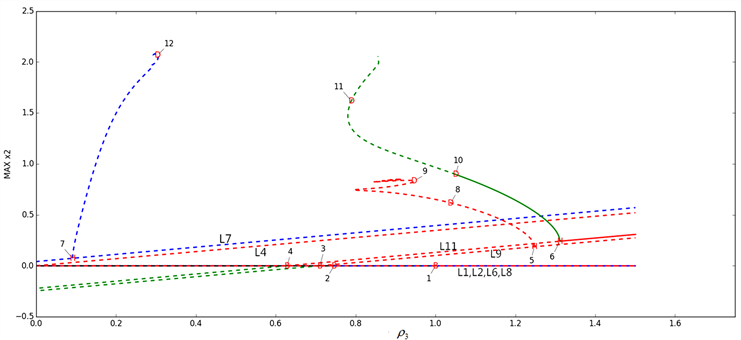Figure 1. The bifurcation diagramTable 1. Bifurcation occured at equilibrium pointsTable 2. Bifurcation generated by periodic solution

${\rho }_{3}$ 从1.31131的右侧减小到1.31131时，系统(3)在 ${P}_{11}$ 处发生了HB (6)；随着 ${\rho }_{3}$ 继续减小，在1.31131的左侧，平衡点 ${P}_{11}$ 的邻域内生成一族稳定的周期轨，如图2所示。当 ${\rho }_{3}$ 减小到1.24771时，系统在 ${P}_{9}$ 处发生了HB (5)，随着 ${\rho }_{3}$ 继续减小，在1.24771的左侧，平点 ${P}_{9}$ 的邻域内生成一族不稳定的周期轨。当 ${\rho }_{3}$ 减小到1.05072时，对应的周期解的4个Floquet乘子为 $\left\{1,-1,0.767665,-4.76211×{10}^{-6}\right\}$，其中一个Floquet乘子在−1处由内而外穿过单位圆导致了PDB (10)的发生，稳定的周期轨失去了稳定性。当 ${\rho }_{3}$ 小于1.05072时，我们模拟出了倍周期分支的级联现象，如图3(a)~(d)所示。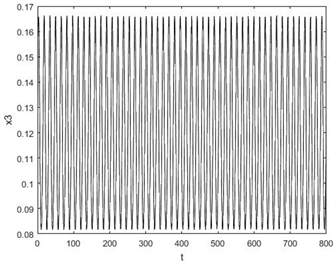Figure 2. The projection of periodic orbit and time series chart of ${x}_{3}$ for ${\rho }_{3}=1.30124$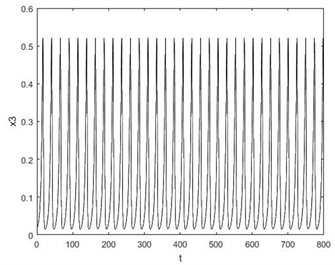(a)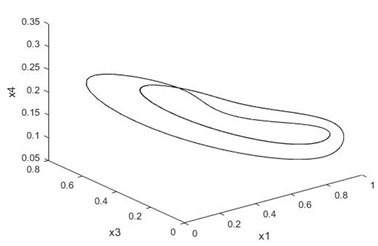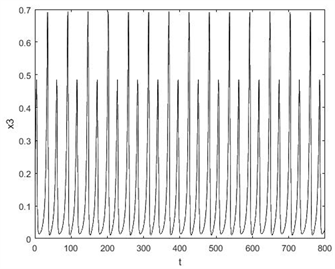(b)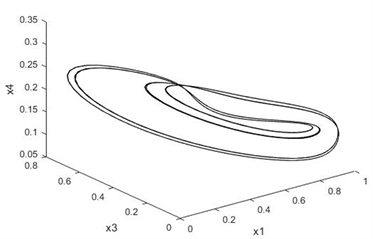(c)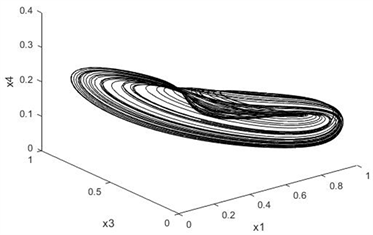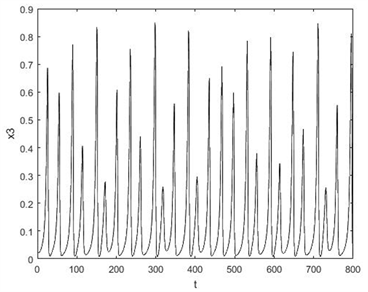(d)

Figure 3. The period-doubling cascade: (a) Period 1 orbit at ${\rho }_{3}=1.1$ ; (b) Period 2 orbit at ${\rho }_{3}=1.03934$ ; (c) Period 4 orbit at ${\rho }_{3}=1.02193$ ; (d) Chaotic attractor at ${\rho }_{3}=1.00196$

${\rho }_{3}=1.00196$ 时，以 $\left(0.\text{68414},0.\text{2469}0,0.0\text{262}0,0.23384\right)$ 为初始点的轨线是一个混沌吸引子，其对应的Lyapunov指数为 $\left(0.0\text{14222},0,-0.0\text{14}0\text{86},-0.\text{44887}\right)$。若 ${\rho }_{3}\in \left(0.947834,1.01265\right)$，系统在 ${P}_{11}$ 邻域内出发的解都是趋于混沌的。

${\rho }_{3}\in \left(0.949534,1\right)$ 时，宿主细胞和免疫细胞的初始数目越多，肿瘤细胞和内皮细胞的初始数目越少，轨线就越容易收敛到无肿瘤平衡点 ${P}_{2}$。当 ${\rho }_{3}<0.949534$ 时，如图5所示，当 ${\rho }_{3}=0.85$${P}_{11}$ 邻域内以 $\left(0.\text{6577},0.\text{24},0.\text{11}0\text{8},0.\text{16}\right)$ 为初始点出发的解随着时间增加会较快地收敛到平衡点 ${P}_{2}$，肿瘤细胞的数量随时间增长趋于0，本文研究的肿瘤细胞的增长率的临界值即为0.949534。模拟结果表明，若肿瘤的增长率通过治疗能降低到临界值以下，肿瘤细胞的数量会在较短时间内衰减至0，其他细胞数量趋于定值。Figure 4. The projection of coexistence of two steady states for ${\rho }_{3}=0.96$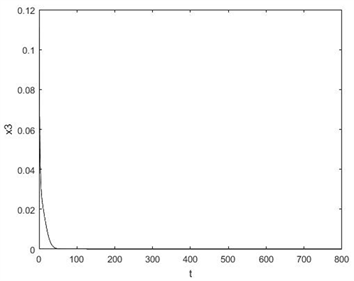Figure 5. The projection of phase diagram for ${\rho }_{3}=0.85$

4. 结论

 Gatenby, R.A. and Maini, P.K. (2003) Mathematical Oncology: Cancer Summed Up. Nature, 421, 321.
https://doi.org/10.1038/421321a

 Kuznetsov, V.A., Makalkin, I.A., Taylor, M.A., et al. (1994) Nonlineardynamics of Immunogenic Tumors: Parameter Estimation and Global Bifurcation Analysis. Bulletin of Mathematical Biology, 56, 295-321.
https://doi.org/10.1016/S0092-8240(05)80260-5

 Pillis, L.G.D. and Radunskaya, A. (2003) The Dynamics of an Optimally Controlled Tumor Model: A Case Study. Mathematical and Computer Modelling, 37, 1221-1244.
https://doi.org/10.1016/S0895-7177(03)00133-X

 Viger, L., Denis, F., Rosalie, M., et al. (2014) A Cancer Model for the Angiogenic Switch. Journal of Theoretical Biology, 360, 21-33.
https://doi.org/10.1016/j.jtbi.2014.06.020

 Stephen, W. and Mazel, D.S. (1990) Introduction to Applied Nonlinear Dynamical Systems and Chaos. Computers in Physics, 4, 563.
https://doi.org/10.1063/1.4822950

Top